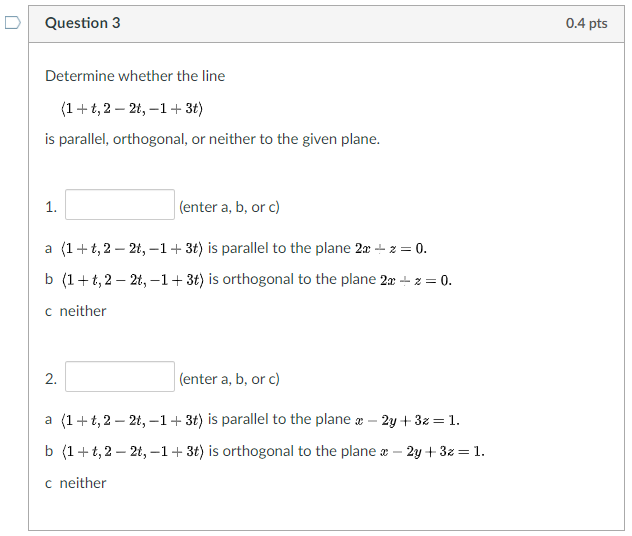# Question Solved1 AnswerQuestion 3 0.4 pts Determine whether the line (1+t, 2 – 2t, -1+ 3t) is parallel, orthogonal, or neither to the given plane. 1. (enter a, b, or c) a (1+t, 2 - 2t, -1+ 3t) is parallel to the plane 2x + =0. b (1+t, 2 – 2t, -1 + 3t) is orthogonal to the plane 22 +2=0. c neither 2. (enter a, b, or c) a (1+t, 2 - 21,-1+ 3t) is parallel to the plane x - 2y+32 = 1. b (1+t, 2 – 2t, -1+ 3t) is orthogonal to the plane x 2y + 3z=1. c neitherTranscribed Image Text: Question 3 0.4 pts Determine whether the line (1+t, 2 – 2t, -1+ 3t) is parallel, orthogonal, or neither to the given plane. 1. (enter a, b, or c) a (1+t, 2 - 2t, -1+ 3t) is parallel to the plane 2x + =0. b (1+t, 2 – 2t, -1 + 3t) is orthogonal to the plane 22 +2=0. c neither 2. (enter a, b, or c) a (1+t, 2 - 21,-1+ 3t) is parallel to the plane x - 2y+32 = 1. b (1+t, 2 – 2t, -1+ 3t) is orthogonal to the plane x 2y + 3z=1. c neither
More
Transcribed Image Text: Question 3 0.4 pts Determine whether the line (1+t, 2 – 2t, -1+ 3t) is parallel, orthogonal, or neither to the given plane. 1. (enter a, b, or c) a (1+t, 2 - 2t, -1+ 3t) is parallel to the plane 2x + =0. b (1+t, 2 – 2t, -1 + 3t) is orthogonal to the plane 22 +2=0. c neither 2. (enter a, b, or c) a (1+t, 2 - 21,-1+ 3t) is parallel to the plane x - 2y+32 = 1. b (1+t, 2 – 2t, -1+ 3t) is orthogonal to the plane x 2y + 3z=1. c neither